Online Tution   »   Class 10   »   Real Numbers

# Real Numbers Class 10 Notes, MCQs, Extra Questions

## Real Numbers Class 10 Notes

R= Real Numbers

Real numbers include both irrational and rational numbers.

I= Integers

Integers are all numbers starting with (…-3, -2, -1, 0, 1, 2, 3…).

Q= Rational Numbers

Rational numbers are real numbers with the pattern p/q, q 0, and p, q I.

• Rational expressions can represent all integers, such as 5 = 5/1.
• Decimal expansion of recurring or non-recurring rational numbers.

Q’ = Irrational Numbers

Real numbers cannot be expressed in the form p/q, whose decimal expansions are non-terminating and non-recurring.

• Roots of primes like √2, √3, √5 etc., are irrational.

N= Natural Numbers

Natural numbers are those that can be counted. N = {1, 2, 3, …}

W= Whole Numbers

Whole numbers are the collective term for zero and all natural numbers. {0, 1, 2, 3,…}

Even Numbers

Even numbers are natural numbers with the form 2n. (2, 4, 6, …}

Odd Numbers

Odd numbers are natural numbers with the form 2n – 1; examples are 1, 3, 5, etc.

• Why can’t the form be expressed as 2n+1?

Remember!

*Natural numbers are exclusively whole numbers.

*Every Whole Number is an integer.

*Rational Numbers are the same as Integers.

*All Rational Numbers are Real Numbers.

Prime Numbers

Prime numbers are all natural numbers bigger than one that can be divided by 1 and the number itself. Examples of prime numbers are 2, 3, 5, 7, and 11.

• Since it only has one factor, 1, it is not a prime number.

Composite Numbers

Composite numbers are any natural numbers that may be divided by one, including the number one itself. Four, six, eight, nine, ten, etc.

• 1 is not a prime number nor a composite number.

## Real Numbers Class 10 Notes- Methods

1. Euclid’s Division Lemma

There are distinct integers q and r that satisfy the equation a = bq + r, 0 r b when given two positive integers a and b.

Observe this: Every time, “r” is lower than “b.” Each “q” and “r” is distinctive.

1. Application Of Lemma

The HCF of two positive numbers is determined using Euclid’s Division Lemma.

Example: How can I find the HCF of 56 and 72?

Steps:-

• Lemma applied to 56 and 72.
• Find “b” and “r” by using a larger number. 72 = 56 × 1 + 16
• Consider 56 as the new dividend and 16 as the new divisor since 16 s not equal to 0. 56 = 16 × 3 + 8
• Once more, if 8 is not equal to 0, apply 16 as the new dividend and 8 as the new divisor. 16 = 8 × 2 + 0

Ace your class 10th board exams with Adda247 live classes for class 10th preparation.

The remainder being zero, (8) is HCF’s divisor

Euclid’s Division Lemma can be extended to all integers except zero, i.e., b 0. This is true even if it is only stated for positive integers.

III. Constructing A Factor Tree

Steps for building a factor tree:

• Put the amount in the form of a prime number plus a composite number.

Example: 48 factorise

• Continue until all primes have been achieved.

Prime factorization of 48 = 24 x 3

1. Fundamental Theorem Of Arithmetic

With the exception of the sequence in which they appear, every composite number can be written as a product of primes, and this expression is singular.

Applications:

• In order to find the HCF and LCM of two or more positive integers.
• Demonstrating the irrationality of numbers
• To identify the type of rational number’s decimal expansion.

https://youtu.be/iVhsSaBB3rI

https://youtu.be/LjKavXs1vfs

#### 1. Finding The HCF And LCM Of Two Or More Positive Numbers Using An Algorithm

Step I: The first step is to factorise each of the positive integers that are given and express each as a product of powers of primes that are arranged in ascending order of prime number.

Step II: To determine HCF, locate the least powerful prime factors and multiply them to obtain HCF.

Step III: Find the largest exponent and multiply it by the exponents to determine the LCM.

#### 2. Demonstrating The Irrationality Of Numbers

• Irrational numbers are those whose sum or difference is not rational.
• An irrational number is the product of a non-zero rational number, or its quotient.

#### 3. To Ascertain How Rational Numbers Expand To Decimal Places:

• Let x = p/q, where p and q are co-primes, represent a rational number with an end to its decimal expansion. When m and n are non-negative integers, the prime factorization of “q” takes the form 2m5n.
• If x = p/q is a rational number with p and q being non-negative integers, and q’s prime factorization does not take the form 2m5n, then x has a non-terminating repeated decimal expansion.

• 23 can be written as: 23 = 2350
• 52 can be written as: 52 = 2052

## Real Numbers For Class 10 Extra MCQ Questions

Ques. The number 7×11×13×15+15 is a

(a) Composite Number

(b) Whole Number

(c) Prime Number

(d) None of these

Ans: (a) and (b) both

Ques. A lemma is an axiom used for providing

(a) other statement

(b) no statement

(d) none of these

Ans: (a) other statement

Ques. Given that HCF (306,657)=9, find LCM (306,657).

Ans: It is given that the HCF of the two numbers 306,657 is 9. We have to find its LCM.

We know that, LCM×HCF = Product of two numbers. Therefore, LCM×HCF= 306×657

LCM= 306×657/HCF

LCM= 306×657/9

LCM=22338

Ques. Prove that 3 + 2√5 is irrational.

Ans: Let 3 + 2√5 be a rational number.

Then the co-primes x and y of the given rational number where (y ≠ 0) is such that:

3 + 2√5 = x/y

Rearranging, we get,

2√5 = (x/y) – 3

√5 = 1/2[(x/y) – 3]

Since x and y are integers, thus, 1/2[(x/y) – 3] is a rational number.

Therefore, √5 is also a rational number. But this confronts the fact that √5 is irrational.

Thus, our assumption that 3 + 2√5 is a rational number is wrong.

Hence, 3 + 2√5 is irrational.

Ques. Check whether 6n can end with the digit 0 for any natural number n.

Ans: If the number 6n ends with the digit zero (0), then it should be divisible by 5, as we know any number with a unit place as 0 or 5 is divisible by 5.

Prime factorization of 6n = (2 × 3)n

Therefore, the prime factorization of 6n doesn’t contain the prime number 5.

Hence, it is clear that for any natural number n, 6n is not divisible by 5 and thus it proves that 6n cannot end with the digit 0 for any natural number n.

Ques. Using Euclid’s Algorithm, find the HCF of 2048 and 960.

Ans. 2048 > 960

Using Euclid’s division algorithm,

2048 = 960 × 2 + 128

960 = 128 × 7 + 64

128 = 64 × 2 + 0

Therefore, the HCF of 2048 and 960 is 64.

Let’s connect via chat or call our senior expert counsellor at +91-9625869989 to learn more about the different streams and options available.

We would love it if we could add some of your insights. If you have a definite goal of scoring the highest marks, then you can resolve your doubts via our app/quizzes and youtube class  assistance (https://youtube.com/c/Adda247Class9and10)

## Real Numbers Class 10 Notes- FAQs

Ques 1. What are the Class 10 real numbers?

Ans. Both rational and irrational numbers are considered to be real numbers.

Ques 2. A real number may not be negative.

Ans. The positive and negative integers, as well as the fractions created from them (also known as rational numbers), as well as the irrational numbers, are all real numbers.

Ques 3. What is the largest divisible number?

Ans. HCF: HCF stands for “Highest Common Factor.” The largest number that may divide the provided integers is what it refers to.

Ques 4. Real numbers have an end?

Ans. There are uncountably infinitely many real numbers, although there are countably infinite natural numbers. The real numbers make up an endless set of numbers that cannot be injectively transferred to the infinite set of natural numbers.

Sharing is caring!

Thank You, Your details have been submitted we will get back to you.
•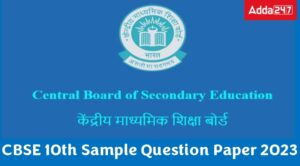CBSE Class 10 Sample Papers 2022-23 for ...
•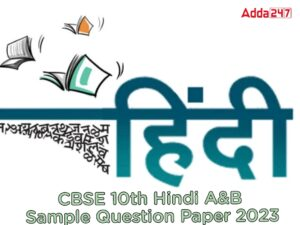CBSE Class 10 Hindi A & B Sample Pap...
•CBSE Class 12 Maths Sample Paper 2022-23...
•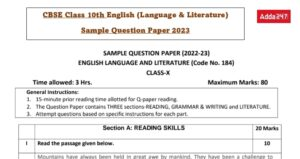Class 10 English Sample Paper 2022- 23 w...
•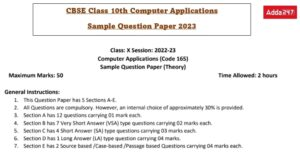CBSE Class 10 Computer Applications Samp...
•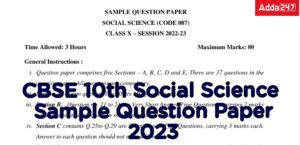Class 10 Social Science (SST) Sample Pap...